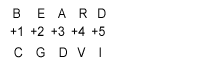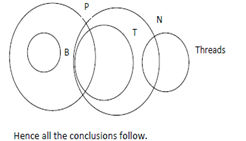# L&T ECC Reasoning Question

1. In a certain code BEARD is written as CGDVI. How is MOUSTACHE written in that code?
1. NQXWYGJPN
2. MQWXJGPN
3. QNWXYGJNP
4. NWXQJGYNP
5. None of these.SimilarlyDIRECTIONS for the question 2: Given an input line; the machine arranges the words and numbers in steps in a systematic manner as illustrated afterwards : Study the pattern and answer the question that follows.
Given an input line, a coding machine rearranges the input following certain steps as explained below:
Input: 47 desert go 56 there often 32 12
Step I: there 47 desert go 56 often 32 12
Step II: there 12 47 desert go 56 often 32
Step III: there 12 often 47 desert go 56 32
Step IV: there 12 often 32 47 desert go 56
Step V: there 12 often 32 go 47 desert 56
2. If Step II of an input is 'waive 14 available time 38 46 probation 85', how many more steps will be required to complete the arrangement?
1. Three
2. Four
3. Five
4. Two
5. None of these
The logic followed in the given sequence is " numbers are arranged in increasing order while words are arranged in decreasing order of the first alphabet "
Step II: waive 14 available time 38 46 probation 85
Step III: waive 14 time available 38 46 probation 85
Step IV: waive 14 time 38 available 46 probation 85
Step V: waive 14 time 38 probation available 46 85
Step VI: waive 14 time 38 probation 46 available 85
Here, step VI is the last step. Hence, four more steps are needed after step II to complete the arrangement.
DIRECTIONS for the questions 3 & 4: The question below contains four statements, followed by four conclusions numbered I, II, III & IV. You have to take the given statements to be true even if they seem to be at variance with commonly known facts. Read all the conclusions and then decide which of the given conclusions logically follows from the given statements disregarding commonly known facts
1. Statements:
a. All books are pins. b. Some pins are tablets. c. All tablets are needles. d. Some needles are
Conclusions:
I. Some needles are pins.
II. Some pins are books.
IV. Some needles are tablets.
1. None follows
2. Only I and II follow
3. Only I, II and III follow
4. Only II, III and IV follow
5. All followI.Clearly, 'Some needles are pins' is true.
II.As 'All books are pins'. So, 'Some pins are books' is true.
III. As 'Some needles are threads', therefore, 'Some threads are needles' is also true.
IV. As 'All tablets are needles' is given, therefore, 'Some needles are tablets' is also true.
Therefore, the correct answer is option E.
2. Statements:
a.All jungles are bins. b. All bins are petals. c.No petal is root. d.All roots are flowers.
Conclusions:
I. No flower is bin.
II. No jungle is root.
III. All jungles are petals.
IV. All flowers are roots.
1. Only I and II follow
2. Only II and III follow
3. Only I, II and III follow
4. All follow
5. None of theseSo, only conclusions II & III follows.
I. No flower is bin : This statement is not necessarily true.
II. No jungle is root : This statement is clearly true.
III. All jungles are petals : This statement is clearly true, as shown in the above figure.
IV. All flowers are roots : This statement can not be true.
Therefore, correct answer is option B.
DIRECTIONS for the questions 5: The question below is followed by two statements marked I and II. Mark as your answer.
1. if the data in statement I alone are suffi­cient to answer the question, while the data in statement II alone are not sufficient to answer the question.
2. if the data in statement II alone are suf­ficient to answer the question, while the data in state­ment I atone are not sufficient to answer the question.
3. if the data either in statement I alone or in statement II alone are sufficient to answer the ques-tion.
4. if the data given in both the statements I and II together are not sufficient to answer the ques­tion; and
5. if the data in both the statements 1 and II together are necessary to answer the question.
3. How is 'steel' written in a code language?
I. 'steel container more costly' is written as 'ho na pa da' in that code language.
II. 'buy more steel vessels' is written as 'na ka ta ja' in that code language.

Statement I alone is not sufficient because it is not certain that the codes of the words are in the same order as the words are in the sentence. Similarly, statement II alone is not sufficient. Now, from I and II: in the code part there must be two codes common in both I and II. But we get only one code 'na' common in both. Thus, the given information is not sufficient to solve the questions.
DIRECTIONS for the questions 6: Solve the following question and mark the best possible option.
'P # Q' means 'P is neither greater than nor equal to Q'.
'P © Q' means' P is neither equal to nor smaller than Q'.
'P % Q' means 'P is neither smaller than nor greater than Q’.
'P \$ Q' means' P is not smaller than Q'.
'P @ Q' means 'P is not greater than Q'.
Now in each of the following questions, assuming the given statements to be true, find which of the three conclu¬sions I, II and III given below them is/are definitely true and give your answer accordingly
4. Statements:
Conclusions:
II. N\$R
1. Only I and II are true
2. Only II and III are true
3. Only I and III are true
4. All are true
5. None of these
Here we have
M > R…(i)
R = D ….(ii)
D < N….(iii)
From (i) and (ii), we get M > R = D…. (iv)
From (ii) and (iii), we get N > D = R….(v)
From (iv) and (v), we do not get any specific relation between M and N. Hence, conclusion I (M > N) does not follow. From (v), we get N > R (conclusion II). Hence, conclusion II follows. Again, from (iv) we get M > D (conclusion III). Hence, conclusion III follows. Hence only II and III are true.
DIRECTIONS for the questions 7-8: Read the information given below and answer the question that follows.
Eight people J, K, L, M, N, O, P and Q are sitting around a circular table, facing the centre, not necessarily in the same order. O is sitting third to the right of M. There is only one person sitting between M and J. There are only three people between J and K. P is an immediate neighbour of J. There are only three people between P and L. N is second to the right of P.
5. Four of the following five are alike in a certain way based on their seating positions in the above arrangement and so form a group. Which does not belong to the group?
1. PQ
2. KL
3. MN
4. QO
5. KO
In all the options except KO the first person is sitting to the right of first. So, answer is KO.
6. What is N's position with respect to K?
1. Second to the left
2. Second to the right
3. Third to the left
4. Third to the right
5. Four to the left
So, N is sitting third to the left of K.
DIRECTIONS for the questions 9 & 10: Solve the following question and mark the best possible option.
a) 'x • y λ z' means 'z is multiplied by the sum of x and y'
b) 'x \$ y % z' means 'y is subtracted from x and the resultant is divided by z;.
c) 'x # @ Y © z' means 'x is added to the resultant when y is divided by z'.
In each of the question below, a set of instruction se¬quences is given. You are required to find out the outcome which should come in place of the question mark (?) in each of the given sets of sequence.
7. I. 9 • 5 λ 15 = n
II. n \$ 20 % 8 = ?
1. 8
2. 40
3. 5
4. 13
5. None of these
we have(1) (9+5) * 15 = 210 = n
Therefore, n = 210;
Now(2) (210-20)/ 8 = 190/ 8 = 23.75 = Hense Option E.
8. I. 26 @ 45 © 9 = k II. k • 14 λ 4 = ?
1. 120
2. 45
3. 49
4. 84
5. None of these